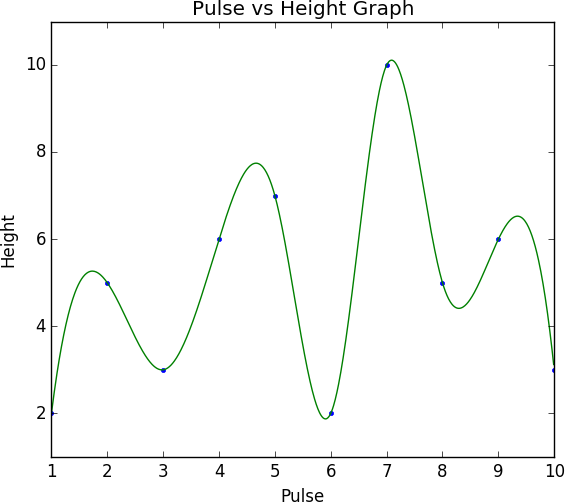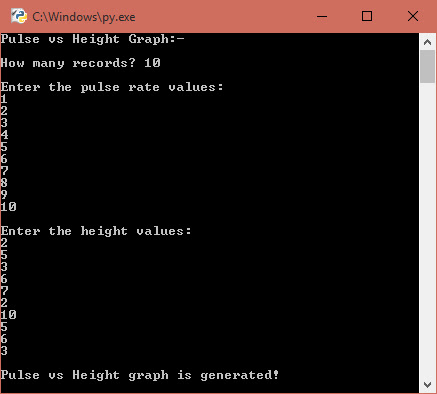### Plotting Graph by Keyboard Input using Python### Introduction to 2D Smooth Graph

Connecting line between two points (x1, y1) and (x2, y2) is known as a graph. A non-uniform cartesian co-ordinates points will generate a rigid line, which looks ugly. In Python, a rigid graph can be regenerated into a smooth graph by interpolating more points using SciPy module. SciPy (Scientific Python library) generates more sub-points based on the input array values to form a smooth curve. In this program, I have used input() function to get n number of points (x, y) from the user through keyboard (screenshot is given in the bottom) and store it to arrays. The co-ordinates points used in the program are (1, 2), (2, 5), (3, 3), (4, 6), (5, 7), (6, 2), (7, 10), (8, 5), (9, 6), and (10, 3).

### Program Implementation

In this tutorial, I have used Python 3.5.2 (64-bit) software, and 7 modules: MatPlotLib 2.0.2, PyParsing 2.2.0, Python-DateUtil 2.6.1, PyTZ 2017.2, SetupTools 36.2.0, Cycler 0.10.0, SciPy 0.19.1, and NumPy-MKL 1.13.1 implemented in Windows 10 Enterprise (64-bit) operating system. The 8 modules are chosen based on the compatibility of Python version and OS bit.

#### Source Code

import scipy.interpolate as inter
import numpy as np
import matplotlib.pyplot as plt

p, h = list(), list()

print("Pulse vs Height Graph:-\n")
n = input("How many records? ")

print("\nEnter the pulse rate values: ")
for i in range(int(n)):
pn = input()
p.append(int(pn))
x = np.array(p)

print("\nEnter the height values: ")
for i in range(int(n)):
hn = input()
h.append(int(hn))
y = np.array(h)

print("\nPulse vs Height graph is generated!")

z = np.arange(x.min(), x.max(), 0.01)
s = inter.InterpolatedUnivariateSpline(x, y)

plt.plot (x, y, 'b.')
plt.plot (z, s(z), 'g-')
plt.xlabel('Pulse')
plt.ylabel('Height')
plt.title('Pulse vs Height Graph')
plt.show()

#### Command Prompt